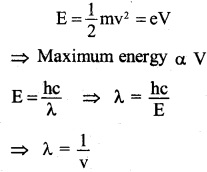# 2nd PUC Physics Question Bank Chapter 12 Atoms

## Karnataka 2nd PUC Physics Question Bank Chapter 12 Atoms

### 2nd PUC Physics Atoms NCERT Text Book Questions and Answers

Question 1.
Choose the correct alternative from the clues given at the end of each statement:

1. The size of the atom in Thomson’s model is ………….. the atomic size in Rutherford’s model, (much greater than/ no different from/much less than.)
2. In the ground state of …………..electrons are in stable equilibrium, while in electrons always experience a net force. (Thomson’s model/ Rutherford’s model.)
3. A classical atom based on ………….. is doomed to collapse. (Thomson’s model/ Rutherford’s model.)
4. An atom has a nearly continuous mass distribution in a ………….. but has a highly non-uniform mass distribution in (Thomson’s model/ Rutherford’s model.)
5. The positively charged part of the atom possesses most of the mass in ………….. (Rutherford’s model/both the models.)

1. No different from
2. Thomson’s model, Rutherford’s model
3. Rutherford’s model
4. Thomson’s model, Rutherford’s model
5. both the models.Question 2
Suppose you are given a chance to repeat the alpha-particle scattering experiment using a thin sheet of solid hydrogen in place of the gold foil. (Hydrogen is a solid at temperatures below 14 K.) What results do you expect?
α-particle is much heavier than hydrogen nuclei. Therefore, α-particles pass through solid hydrogen without deflection. In other words, α-particles are not scattered by solid hydrogen.

Question 3.
What is the shortest wavelength present in the Paschen series of spectral lines?
The wavelengths of the spectral lines in the Paschen series are given byQuestion 4.
A difference of 2.3 eV separates two energy levels in an atom. What is the frequency of radiation emitted when the atom make a transition from the upper level to the lower level?Question 5.
The ground state energy of the hydrogen atom is -13.6 eV. What are the kinetic and potential energies Of the electron in this state?
The kinetic energy of the electron in an orbit is numerically equal to its total energy. Therefore, the kinetic energy of the electron = 13.6 eV
The potential energy of the electron in an orbit is equal to twice, its total energy
= -13.6 ×2
= -27.2 eV.

Question 6.
A hydrogen atom initially in the ground level absorbs a photon, which excites it to the n = 4 level. Determine the wavelength and frequency of the photon.
The energy of the electron in the ground state
Ee = -13.6 eV
Also, the energy of the electron in n=4 level,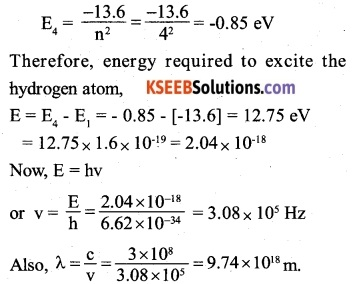Question 7.
(a) Using Bohr’s model calculates the speed of the electron in a hydrogen atom in the n = 1, 2, and 3 levels,
(b) Calculate the orbital period in each of these levels.
(a) The speed of an electron in the nth orbit of an H-atom is given byFrom equation (i), it follows that the velocity of electron in an orbit of a hydrogen atom is inversely proportional to its quantum number (n).(b) The orbital period of an electron in the nth orbit of an H-atom is given by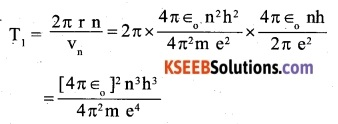It follows that orbital period of an electron in H-atom is directly proportional to the cube of its quantum number.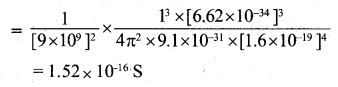Question 8.
The radius of the innermost electron orbit of a hydrogen atom is 5.3 x 10-11. What are the radii of the n = 2 and n = 3 orbits?
The radius of an orbit in a hydrogen atom is directly proportional to the square of its quantum number i.e.Question 9.
A 12.5 eV electron beam is used to bombard gaseous hydrogen at room temperature. What series of wavelengths will be emitted?
The first, second and third excited energies for hydrogen atom are
E’ 1 = E2 – E1 = -3.4 – [-13.6] = 10.2 eV,
E’2 = E3 – E1= -1.51 – [-13.6] = 12.09 eV,
and E’3 = E4 – E1 = -0.85 – [-13.6] = 12.75 eV
It follows that when a 12.5 eV electron beam is used to bombard gaseous hydrogen at room temperature, the H – atoms at the most will be raised to n = 3 level. The H – atoms may come to ground state directly or via n = 2 level. Therefore, the wavelengths emitted will lie in Balmer series [for de-excitation from n = 3 level to n = 2 and 1 level] and Lyman series [for de­excitation from n = 2 level to n = 1 level].Question 10.
In accordance with the Bohr’s model, find the quantum number that characterises the earth’s revolution around the sun in an orbit of radius  1.5 x 1011 m with orbital speed 3 x 104 m/s. (Mass of earth = 6.0 x 1024)
Here, m = 6.0 x 1024 kg; r = 1.5×10nm
and v = 3 x 104 ms-1
The angular momentum of the earth,
mvr = 6.0×1024 x 3 x 104 x 1.5 x 1011 = 2.7 x 1040 kg ms-1
In accordance with Bohr’s model### 2nd PUC Physics Atoms Additional Exercise

Question 11.
Answer the following questions, which help you understand the difference between Thomson’s model and Rutherford’s model better.

(a) Is the average angle of deflection of α-particles by a thin gold foil predicted by Thomson’s model much less, about the same, or much greater than that predicted by Rutherford’s model?

(b) Is the probability of backward scattering (i.e., scattering of α-particles at angles greater than 90°) predicted by Thomson’s model much less, about the same, or much greater than that predicted by Rutherford’s model?

(c) Keeping other factors fixed, it is found experimentally that for small thickness t, the number of α-particles scattered at moderate angles is proportional to t. What clue does this linear dependence on t provide?

(d) In which model is it completely wrong to ignore multiple scattering for the calculation of average angle of scattering of a -particles by a thin foil?

(a) Is the average angle of deflection of α-particles by a thin gold foil predicted by Thomson’s model much less, about the same, or much greater than that predicted by Rutherford’s model?

(b) Is the probability of backward scattering (i.e., scattering of α-particles at angles greater than 90°) predicted by Thomson’s model much less, about the same, or much greater than that predicted by Rutherford’s model?

(c) Keeping other factors fixed, it is found experimentally that for small thickness t, the number of α-particles scattered at moderate angles is proportional to t. What clue does this linear dependence on t provide?

(d) In which model is it completely wrong to ignore multiple scattering for the calculation of the average angle of scattering of a -particles by a thin foil?Question 12.
The gravitational attraction between electron and proton in a hydrogen atom is weaker than the Coulomb attraction by a factor of about 10-40. An alternative way of looking at this fact is to estimate the radius of the first Bohr orbit of a hydrogen atom if the electron and proton were bound by gravitational attraction. You will find the answer interesting.
Let m and m be the masses of electron and proton respectively. If the electron and proton were bound only by gravitational attraction, then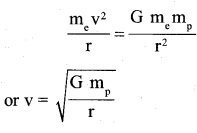According to Bohr’s quantization conditions, the angular momentum of the electron.Question 13.
Obtain an expression for the frequency of radiation emitted when a hydrogen atom de-excites from level n to level (n-1). For large n, show that this frequency equals the classical frequency of revolution of the electron in the orbit.
When a hydrogen atom de-excites from level n1 to np the frequency of radiation emitted is given by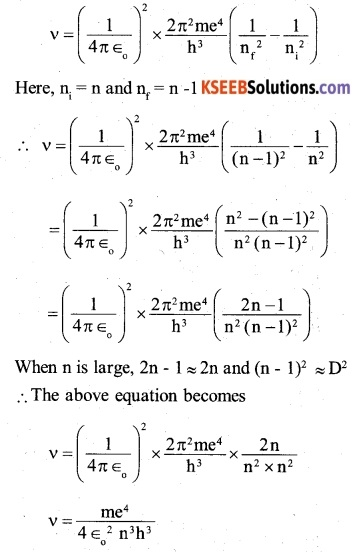Hence, when n is large, the frequency of radiation emitted is equal to the classical frequency of revolution of the electron in the orbit.Question 14.
Classically, an electron can be in any orbit around the nucleus of an atom. Then what determines the typical atomic size? Why is an atom not, say, a thousand times bigger than its typical size? The question had greatly puzzled Bohr before he arrived at his famous model of the atom that you have learned in the text. To simulate what he might well have done before his discovery, let us play as follows with the basic constants of nature and see if we can get a quantity with the dimensions of length that is roughly equal to the known size of an atom (~ 10-10m).

(a) Construct a quantity with the dimensions of length from the fundamental constants e, me, and c. Determine its numerical value.

(b) You will find that the length obtained in (a) is many orders of magnitude smaller than the atomic dimensions. Further, it involves c. But energies of atoms are mostly in non-relativistic domain where c is not expected to play any role. This is what may have suggested Bohr to discard c and look for ‘something else’ to get the right atomic size. Now, the Planck’s constant h had already made its appearance elsewhere. Bohr’s great insight lay in recognising that h, me, and e will yield the right atomic size. Construct a quantity with the dimension of length from h, me, and e and confirm that its numerical value has indeed the correct order of magnitude.
(a) The quantity with the dimensions of length constructed from the fundamental constants e, m, and c is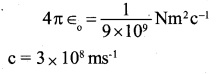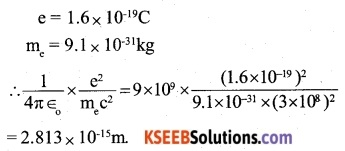(b) The quantity with the dimensions of length constructed from the fundamental constants h, e, and m is the radius of the innermost orbit (n = 1) of the H – atom. It is given by,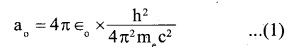It is also called Bohr’s radius
Substituting the values of h, me and 4π∈o in equation (1),Question 15.
The total energy of an electron in the first excited state of the hydrogen atom is about -3.4 eV.
(a) What is the kinetic energy of the electron in this state?
(b) What is the potential energy of the electron in this state?
(c) Which of the answers above would change if the choice of the zero of potential energy is changed?
We know that K.E and P.E of the electron in the nth energy state of the hydrogen atom are respectively given by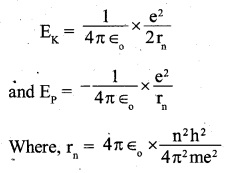The first excited state corresponds to n = 2 level.
Now, the total energy of an electron in
n = 2 state is given to be – 3.4 eV.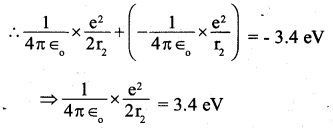(a) Therefore, K.E of the electron in the first excited state (n = 2) of the hydrogen atom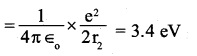(b) E of the electron in the first excited state (n = 2) of the hydrogen atom.(c) If the zero of potential energy is chosen differently, K.E does not change. The P.E and total energy will changeQuestion 16.
If Bohr’s quantisation postulate (angular momentum = nh/2π) is a basic law of nature, it should be equally valid for the case of planetary motion also. Why then do we never speak of quantisation of orbits of planets around the sun?
The mass of a planet is very large as compared to that of electron and accordingly, its angular momentum is very large. For $$\frac { h }{ 2\pi }$$ example, in terms of the angular, from the
Bohr’s quantisation relation,$$\frac { nh }{ 2\pi }$$ it follows that for the orbital motion of the earth, n = 1070. For such a large value of n, the difference in the energies of the successive energy levels are so small as compared to the energies associated with the energy levels that these levels form an energy continuum, i.e the levels appear to be continuous.

Question  17.
Obtain the first Bohr’s radius and the ground state energy of a muonic hydrogen atom [i.e., an atom in which a negatively charged muon (μ) of mass about 207me orbits around a proton].
Let m be the mass of the electron. the firest Bohr radius of H- atom is given by,in a muonic hydrogen atom, in place of an electron, a negatively charged muon having mass 207 m. revolves around the nucleus of hydrogen i.e. proton.
If r1‘ is first Bohr’s radius of muonic hydrogen atom,Question 1.
What is the ratio of radii of the orbits corresponding to first excited state and ground state of hydrogen atom?
The radius of the nth orbit, rn α n2
The ground state corresponds to n = 1 and the
a first excited state corresponds to n = 2Question 2.
The ground state energy of the hydrogen atom is -13.6eV. What are the kinetic and potential energies of the electron in this state?
The potential energy (Ep) of the electron in an orbit is equal to twice its total energy (E) = -13.6 x 2 = -27.2eV
The kinetic energy (Ek) of the electron in an orbit is equal to the negative of its total energy (E) = Ek – E = -(-13.6) = 13.6 eV.

Question 3.
The radius of 1st electron orbit of hydrogen atom is 5.3 x 10-11 m. What is the radius of 2nd orbit?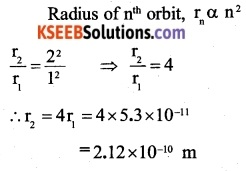Question 4.
What is energy possessed by an electron in n = ∞ ?
Energy of electron in nth orbit of a hydrogen atom is given by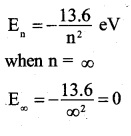Question 5.
If 13.6eV energy is required to ionise the hydrogen atom, then the energy required to remove an electron from n = 2 is
(A) 2 eV
(B) 0 eV
(C) 3.4 eV
(D) 6.8 eV
Required energy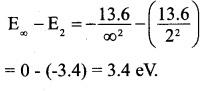Question 6.
The transition from state n = 3 to n = 1 in a hydrogen like atom results in ultraviolet radiation. Infrared radiation will be obtained in transition from
(A) n = 2  to  n = 1
(B) n = 3 to n = 2
(C) n = 4  to n = 2
(D) n = 5 to n = 4
Infrared region lies for the Bracket series, i.e. the transition from n = 5 to n = 4.

Question 7.
Which of the following transition in a hydrogen atom emits the photon of highest frequency?
(A) n = 2 to  n = 6
(B) n   = 2 to n =   1
(C) n = 6  to    n = 2
(D) n   = 1 to n =   2
$$v={ R }_{ c }\left( \frac { 1 }{ { n }_{ i }^{ 2 } } -\frac { 1 }{ { n }_{ f }^{ 2 } } \right)$$
Substituting various values of n and np it is observed, maximum frequency is obtained for transition from n = 2 to n = 1.

Question 8.
When a hydrogen atom is raised from ground to excited state,
(A) The P.E increases and K.E decreases
(B) The P.E decreases and K.E increases
(c) Both P.E and K.E increase
(d) Both P.E and K.E decreases
(B) The P.E decreases and K.E increasesQuestion 9.
In which of these, will radius of first orbit be minimum?
(A) H atom
(B) 21H atom
(C) He+ ion
(D) Li2+ ion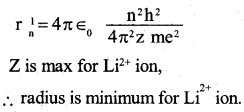(A) $$\sqrt { v }$$
(B) $${ v }^{ 2 }$$
(C) $$\frac { 1 }{ \sqrt { v } }$$
(D) $$\frac { 1 }{ v }$$
(D) $$\frac { 1 }{ v }$$